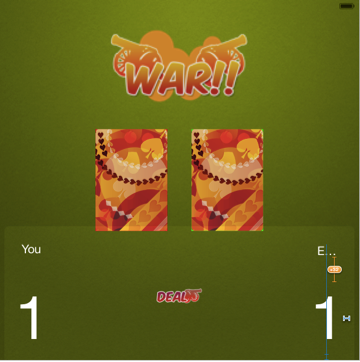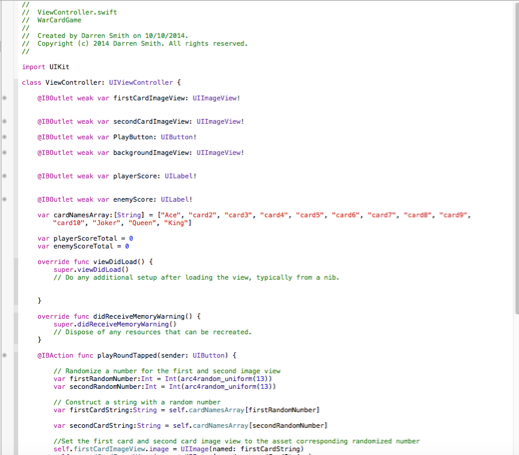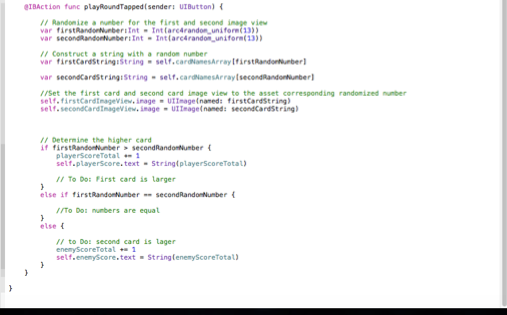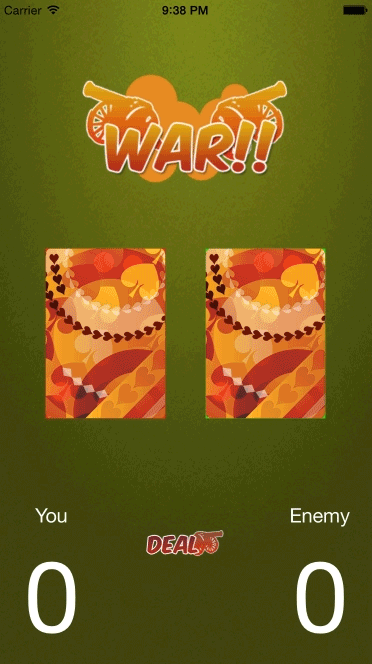# Xcode Swift Code: Implementing the Score and the Finish touches to the Card Game

Xcode Swift Code: Implementing the Score and the Finish touches to the Card Game

Today I added finishing touches to the card game. The background was changed and the score text is implemented as shown in figure 1 below.Figure 1: Adding the Player and Enemy Score Text

The next step is to implement the scoring system into the game. This is done by adding the Player and Enemy variables. What these variables do is they store the integer would be in this case the score data. This is illustrated in figure 2 below.Figure 2: Enemy and Player Variables

Once the variables are added, the scoring conditions are then added into the if statement that is already created. What this condition does is if the player draws a card which is a higher value than the card that enemy has drawn, then he will score a point. However if the cards drawn are both the same then nothing happens and lastly if the enemy draws a card which is higher than the cad that the player has drawn, then he will gain a point. Below in figure 3 is the implementation of the scoring conditions and in figure 4 is the result end of the code.Figure 3: Setting the Scoring ConditionsFigure 4: End Result!